# quantumComputers (1).ppt

31 de May de 2023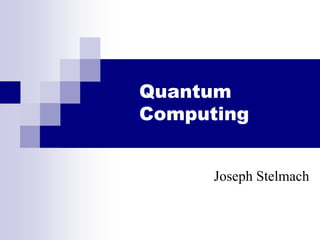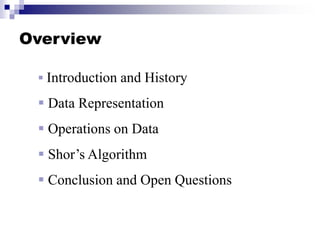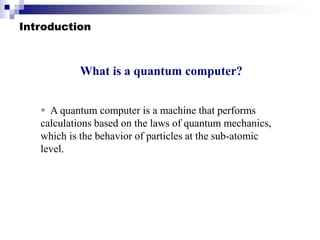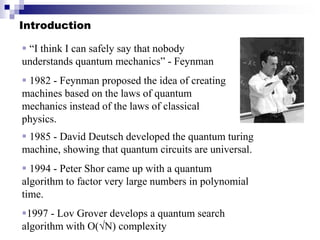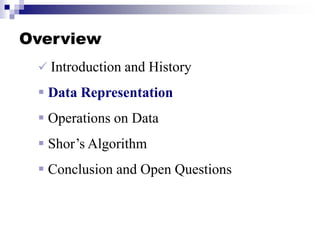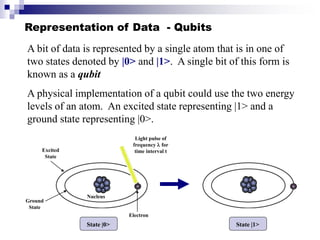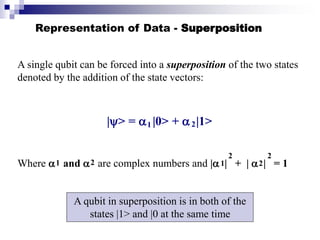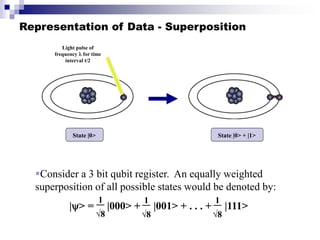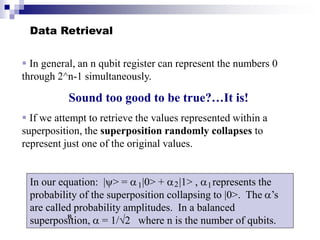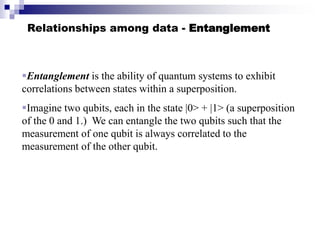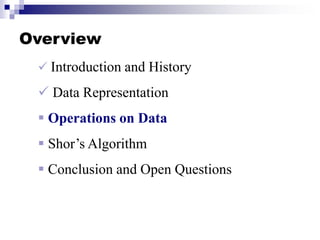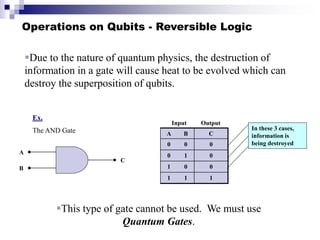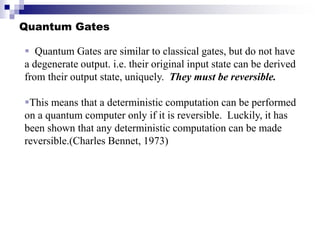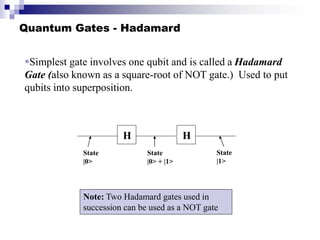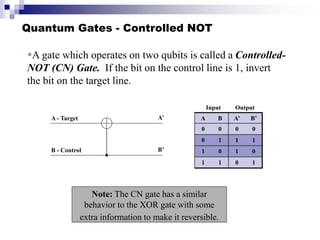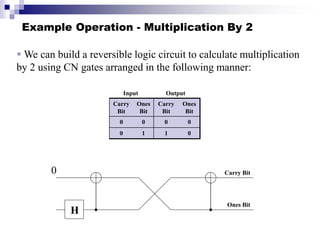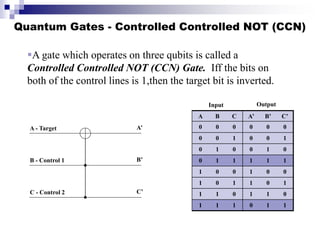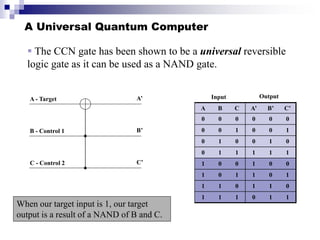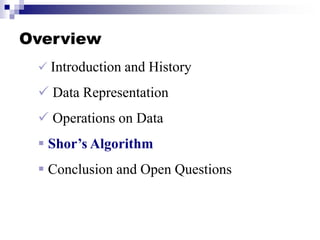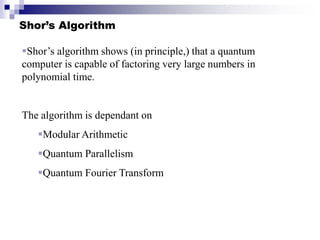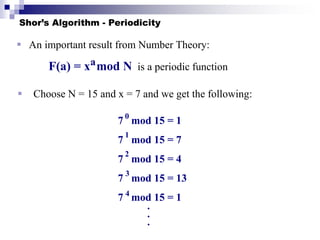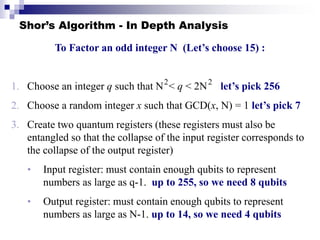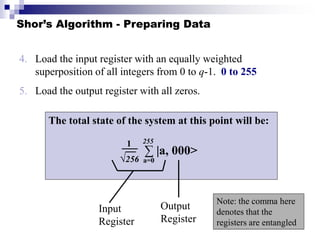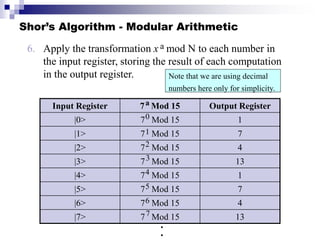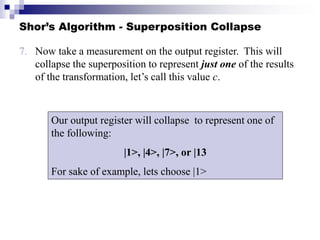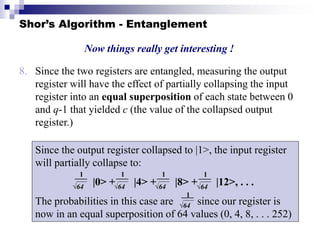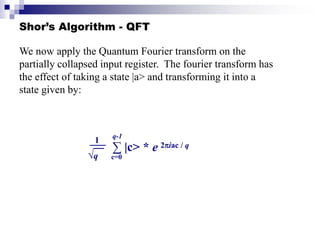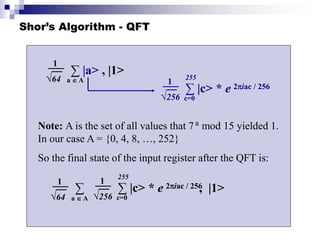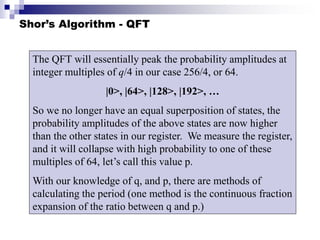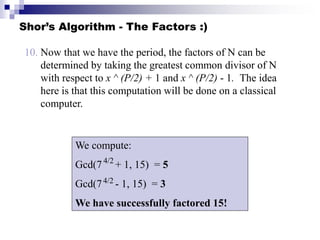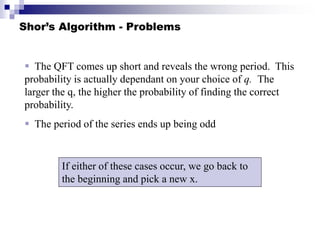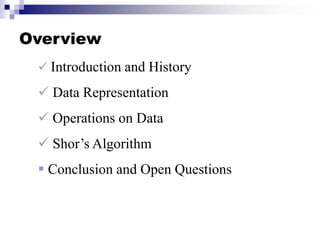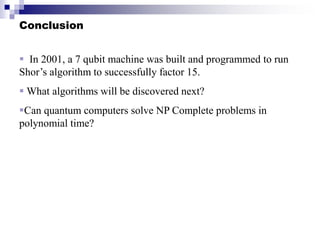1 de 33

### quantumComputers (1).ppt

• 1. Quantum Computing Joseph Stelmach
• 2. Overview  Introduction and History  Data Representation  Operations on Data  Shor’s Algorithm  Conclusion and Open Questions
• 3. Introduction What is a quantum computer?  A quantum computer is a machine that performs calculations based on the laws of quantum mechanics, which is the behavior of particles at the sub-atomic level.
• 4. Introduction  “I think I can safely say that nobody understands quantum mechanics” - Feynman  1982 - Feynman proposed the idea of creating machines based on the laws of quantum mechanics instead of the laws of classical physics.  1985 - David Deutsch developed the quantum turing machine, showing that quantum circuits are universal.  1994 - Peter Shor came up with a quantum algorithm to factor very large numbers in polynomial time. 1997 - Lov Grover develops a quantum search algorithm with O(√N) complexity
• 5. Overview  Introduction and History  Data Representation  Operations on Data  Shor’s Algorithm  Conclusion and Open Questions
• 6. Representation of Data - Qubits A bit of data is represented by a single atom that is in one of two states denoted by |0> and |1>. A single bit of this form is known as a qubit A physical implementation of a qubit could use the two energy levels of an atom. An excited state representing |1> and a ground state representing |0>. Excited State Ground State Nucleus Light pulse of frequency  for time interval t Electron State |0> State |1>
• 7. Representation of Data - Superposition A single qubit can be forced into a superposition of the two states denoted by the addition of the state vectors: |> =  |0> +  |1> Where  and  are complex numbers and | | + |  | = 1 1 2 1 2 1 2 2 2 A qubit in superposition is in both of the states |1> and |0 at the same time
• 8. Representation of Data - Superposition Light pulse of frequency  for time interval t/2 State |0> State |0> + |1> Consider a 3 bit qubit register. An equally weighted superposition of all possible states would be denoted by: |> = |000> + |001> + . . . + |111> 1 √8 1 √8 1 √8
• 9. Data Retrieval  In general, an n qubit register can represent the numbers 0 through 2^n-1 simultaneously. Sound too good to be true?…It is!  If we attempt to retrieve the values represented within a superposition, the superposition randomly collapses to represent just one of the original values. In our equation: |> =  |0> +  |1> ,  represents the probability of the superposition collapsing to |0>. The ’s are called probability amplitudes. In a balanced superposition,  = 1/√2 where n is the number of qubits. 1 2 1 n
• 10. Relationships among data - Entanglement Entanglement is the ability of quantum systems to exhibit correlations between states within a superposition. Imagine two qubits, each in the state |0> + |1> (a superposition of the 0 and 1.) We can entangle the two qubits such that the measurement of one qubit is always correlated to the measurement of the other qubit.
• 11. Overview  Introduction and History  Data Representation  Operations on Data  Shor’s Algorithm  Conclusion and Open Questions
• 12. Due to the nature of quantum physics, the destruction of information in a gate will cause heat to be evolved which can destroy the superposition of qubits. Operations on Qubits - Reversible Logic A B C 0 0 0 0 1 0 1 0 0 1 1 1 Input Output A B C In these 3 cases, information is being destroyed Ex. The AND Gate This type of gate cannot be used. We must use Quantum Gates.
• 13. Quantum Gates  Quantum Gates are similar to classical gates, but do not have a degenerate output. i.e. their original input state can be derived from their output state, uniquely. They must be reversible. This means that a deterministic computation can be performed on a quantum computer only if it is reversible. Luckily, it has been shown that any deterministic computation can be made reversible.(Charles Bennet, 1973)
• 14. Quantum Gates - Hadamard Simplest gate involves one qubit and is called a Hadamard Gate (also known as a square-root of NOT gate.) Used to put qubits into superposition. H State |0> State |0> + |1> H State |1> Note: Two Hadamard gates used in succession can be used as a NOT gate
• 15. Quantum Gates - Controlled NOT A gate which operates on two qubits is called a Controlled- NOT (CN) Gate. If the bit on the control line is 1, invert the bit on the target line. A - Target B - Control A B A’ B’ 0 0 0 0 0 1 1 1 1 0 1 0 1 1 0 1 Input Output Note: The CN gate has a similar behavior to the XOR gate with some extra information to make it reversible. A’ B’
• 16. Example Operation - Multiplication By 2 Carry Bit Carry Bit Ones Bit Carry Bit Ones Bit 0 0 0 0 0 1 1 0 Input Output Ones Bit  We can build a reversible logic circuit to calculate multiplication by 2 using CN gates arranged in the following manner: 0 H
• 17. Quantum Gates - Controlled Controlled NOT (CCN) A - Target B - Control 1 C - Control 2 A B C A’ B’ C’ 0 0 0 0 0 0 0 0 1 0 0 1 0 1 0 0 1 0 0 1 1 1 1 1 1 0 0 1 0 0 1 0 1 1 0 1 1 1 0 1 1 0 1 1 1 0 1 1 Input Output A’ B’ C’ A gate which operates on three qubits is called a Controlled Controlled NOT (CCN) Gate. Iff the bits on both of the control lines is 1,then the target bit is inverted.
• 18. A Universal Quantum Computer  The CCN gate has been shown to be a universal reversible logic gate as it can be used as a NAND gate. A - Target B - Control 1 C - Control 2 A B C A’ B’ C’ 0 0 0 0 0 0 0 0 1 0 0 1 0 1 0 0 1 0 0 1 1 1 1 1 1 0 0 1 0 0 1 0 1 1 0 1 1 1 0 1 1 0 1 1 1 0 1 1 Input Output A’ B’ C’ When our target input is 1, our target output is a result of a NAND of B and C.
• 19. Overview  Introduction and History  Data Representation  Operations on Data  Shor’s Algorithm  Conclusion and Open Questions
• 20. Shor’s Algorithm Shor’s algorithm shows (in principle,) that a quantum computer is capable of factoring very large numbers in polynomial time. The algorithm is dependant on Modular Arithmetic Quantum Parallelism Quantum Fourier Transform
• 21. Shor’s Algorithm - Periodicity  Choose N = 15 and x = 7 and we get the following: 7 mod 15 = 1 7 mod 15 = 7 7 mod 15 = 4 7 mod 15 = 13 7 mod 15 = 1 0 1 2 3 4  An important result from Number Theory: F(a) = x mod N is a periodic function a . . .
• 22. Shor’s Algorithm - In Depth Analysis To Factor an odd integer N (Let’s choose 15) : 1. Choose an integer q such that N < q < 2N let’s pick 256 2. Choose a random integer x such that GCD(x, N) = 1 let’s pick 7 3. Create two quantum registers (these registers must also be entangled so that the collapse of the input register corresponds to the collapse of the output register) • Input register: must contain enough qubits to represent numbers as large as q-1. up to 255, so we need 8 qubits • Output register: must contain enough qubits to represent numbers as large as N-1. up to 14, so we need 4 qubits 2 2
• 23. Shor’s Algorithm - Preparing Data 4. Load the input register with an equally weighted superposition of all integers from 0 to q-1. 0 to 255 5. Load the output register with all zeros. The total state of the system at this point will be: 1 √256 ∑ |a, 000> a=0 255 Input Register Output Register Note: the comma here denotes that the registers are entangled
• 24. Shor’s Algorithm - Modular Arithmetic 6. Apply the transformation x mod N to each number in the input register, storing the result of each computation in the output register. a Input Register 7 Mod 15 Output Register |0> 7 Mod 15 1 |1> 7 Mod 15 7 |2> 7 Mod 15 4 |3> 7 Mod 15 13 |4> 7 Mod 15 1 |5> 7 Mod 15 7 |6> 7 Mod 15 4 |7> 7 Mod 15 13 a 0 1 7 6 5 4 3 2 Note that we are using decimal numbers here only for simplicity. . .
• 25. Shor’s Algorithm - Superposition Collapse 7. Now take a measurement on the output register. This will collapse the superposition to represent just one of the results of the transformation, let’s call this value c. Our output register will collapse to represent one of the following: |1>, |4>, |7>, or |13 For sake of example, lets choose |1>
• 26. Shor’s Algorithm - Entanglement 8. Since the two registers are entangled, measuring the output register will have the effect of partially collapsing the input register into an equal superposition of each state between 0 and q-1 that yielded c (the value of the collapsed output register.) Now things really get interesting ! Since the output register collapsed to |1>, the input register will partially collapse to: |0> + |4> + |8> + |12>, . . . The probabilities in this case are since our register is now in an equal superposition of 64 values (0, 4, 8, . . . 252) 1 √64 1 √64 1 √64 1 √64 1 √64
• 27. Shor’s Algorithm - QFT We now apply the Quantum Fourier transform on the partially collapsed input register. The fourier transform has the effect of taking a state |a> and transforming it into a state given by: 1 √q ∑ |c> * e c=0 q-1 2iac / q
• 28. Shor’s Algorithm - QFT 1 √256 ∑ |c> * e c=0 255 2iac / 256 1 √64 ∑ |a> , |1> a  A Note: A is the set of all values that 7 mod 15 yielded 1. In our case A = {0, 4, 8, …, 252} So the final state of the input register after the QFT is: a 1 √64 ∑ , |1> a  A 1 √256 ∑ |c> * e c=0 255 2iac / 256
• 29. Shor’s Algorithm - QFT The QFT will essentially peak the probability amplitudes at integer multiples of q/4 in our case 256/4, or 64. |0>, |64>, |128>, |192>, … So we no longer have an equal superposition of states, the probability amplitudes of the above states are now higher than the other states in our register. We measure the register, and it will collapse with high probability to one of these multiples of 64, let’s call this value p. With our knowledge of q, and p, there are methods of calculating the period (one method is the continuous fraction expansion of the ratio between q and p.)
• 30. Shor’s Algorithm - The Factors :) 10. Now that we have the period, the factors of N can be determined by taking the greatest common divisor of N with respect to x ^ (P/2) + 1 and x ^ (P/2) - 1. The idea here is that this computation will be done on a classical computer. We compute: Gcd(7 + 1, 15) = 5 Gcd(7 - 1, 15) = 3 We have successfully factored 15! 4/2 4/2
• 31. Shor’s Algorithm - Problems  The QFT comes up short and reveals the wrong period. This probability is actually dependant on your choice of q. The larger the q, the higher the probability of finding the correct probability.  The period of the series ends up being odd If either of these cases occur, we go back to the beginning and pick a new x.
• 32. Overview  Introduction and History  Data Representation  Operations on Data  Shor’s Algorithm  Conclusion and Open Questions
• 33. Conclusion  In 2001, a 7 qubit machine was built and programmed to run Shor’s algorithm to successfully factor 15.  What algorithms will be discovered next? Can quantum computers solve NP Complete problems in polynomial time?